# Shapes

Polygon– Simple closed figures having three or more line segment are called polygon (or simple closed curve).

Triangle– A three sided polygon is called triangle.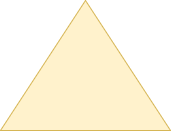Pentagon– A five sided polygon is called a pentagon.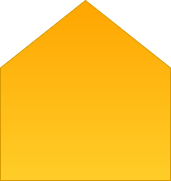Hexagon– A six sided polygon is called hexagon.Heptagon– A seven sided polygon is called heptagon.

Octagon– A eight sided polygon is called octagon.
Nonagon– A nine sided polygon is called nonagon.
Decogon– A ten sided polygon is called decogon.
Regular polygon– It’s all side are equal & all angles are also equal then it is called a regular polygon.
Angle– An angle is a figure formed by two rays with the common initial point. The common initial point is called the vertex of the angle & the rays forming the angle are called its arm.
An angle can also be describe by rotating a ray.
Where OA is initial position and it rotate at center O to final position OB.
Then AOB is an angle, O is vertex and OA and OB are sides of angle.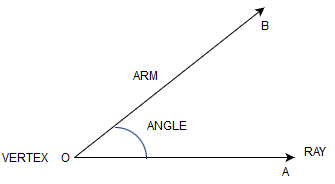Right angle– If the initial position of a ray is horizontal & it rotates to occupy vertical position then the angle formed is called a right angles(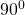).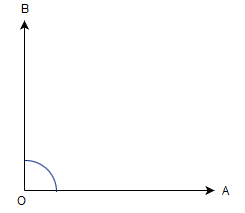Straight angle– If the initial & the final position of a rotating ray are opposite to each other the angle formed is called a straight angle.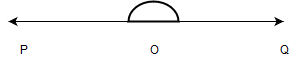Complete angle– If a rotating ray after a complete revolution considers with the initial position the angle formed is called a complete angle.
Adjacent angle– Two angles are said to be adjacent, if they have a common vertex, arm and other two arms are on the opposite side of the common arm.Kinds of angle:-

Acute angle– An angle whose measure is greater than but less than.
Obtuse angle-An angle which is greater than a right angle. But less than straight angle (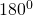) is called obtuse angle.
Reflex angle– An angle whose measure is more than 1800 but less than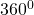, is called reflex angle.
Right angle– An angle whose measure is.